# A No. 10 steel rebar is tested in tension. By monitoring the load reading of the testing machine, it was found that the specimen yielded at a load of 41,600 lb and fractured at 48,300 lb.a. Determine the tensile stresses at yield and at fracture.b. Estimate how much increase in length would occur at 70% of the yield stress in a 2-in. gauge length.

Question
1 views

A No. 10 steel rebar is tested in tension. By monitoring the load reading of the testing machine, it was found that the specimen yielded at a load of 41,600 lb and fractured at 48,300 lb.
a. Determine the tensile stresses at yield and at fracture.
b. Estimate how much increase in length would occur at 70% of the yield stress in a 2-in. gauge length.

check_circle

Step 1

For No.10 rebar, Area = 1.27 in2.

It is required to determine the tensile stress at yield.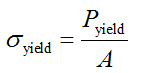Here, Pyield is the load at load and A is the area of the rebar.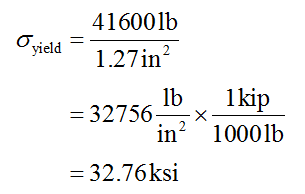Step 2

It is required to determine the tensile stress at fracture.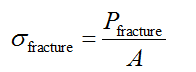Here, Pfracture is the load at load and A is the area of the rebar.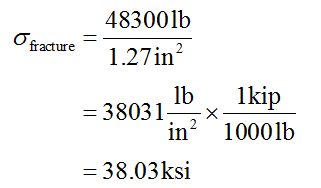Step 3

It is required to determine the increase in length occurs at 70% of yield stress.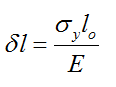Here, lo is the original length, E is the modulus of elasticity. Generally for steel E = 29000 ksi.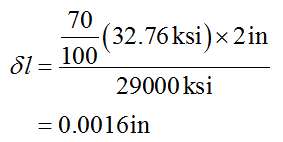...

### Want to see the full answer?

See Solution

#### Want to see this answer and more?

Solutions are written by subject experts who are available 24/7. Questions are typically answered within 1 hour.*

See Solution
*Response times may vary by subject and question.
Tagged in